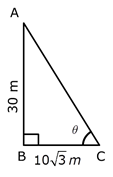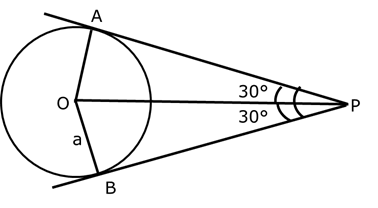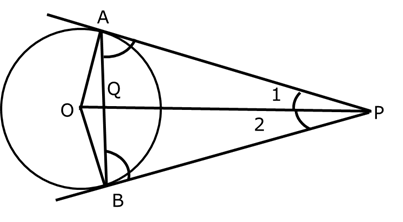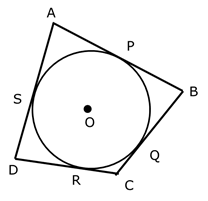# CBSE[All India]_X_Mathematics_2017_Set II

To Access the full content, Please Purchase

• Q1

If a tower 30 m high, casts a shadow  m long on the ground, then what is the angle of elevation of the sun?

Marks:1

Let the tower AB has height of 30 m and length of its shadow
be 10√3 m. Let the angle of elevation of sun be θ
.Therefore, the angle of elevation of the sun is 60°.

• Q2

The probability of selecting a rotten apple randomly from a heap of 900 apples is 0.18. what is the number of rotten apples in the heap?

Marks:1

Number of apples in heap = 900
P(a rotten apple) = 0.18

Let number of rotten apple = x• Q3

What is the common difference of an A.P. in which a21–a7= 84?

Marks:1

Let first term and common difference of A.P. are a and d respectively.

Given; A21 – A7 = 84

(a + 20d) – (a + 6d) = 84

20d – 6d = 84

14d = 84

d = 84/14
= 6

Therefore, the common difference is 6.

• Q4

If the angle between two tangents drawn from an external point P to a circle of radius a and centre O, is 60°, then find the length of OP.

Marks:1

Since, OP is the bisector of angle APB and radius of circle is a.

So, angle APO = 30°.• Q5

A line intersects the y-axis and x-axis at the points P and Q respectively. If (2,-5) is the mid-point of PQ, then find the coordinates of P and Q.

Marks:2

• Q6

If the distances of P(x, y) from A(5, 1) and B(-1, 5) are equal, then prove that 3x = 2y.

Marks:2

• Q7

Find the value of p, for which one root of the quadratic equation px2 – 14x + 8 = 0 is 6 times the other.

Marks:2

• Q8

Prove that the tangents drawn at the end points of a chord of a circle make equal angles with the chord.

Marks:2• Q9

A circle touches all the four sides of a quadrilateral ABCD,   Prove that AB + CD  = BC + DA

Marks:2Since, the tangents drawn on a circle from same external point are equal.

AP = AS

BP = BQ

CQ = CR

DR = DS

LHS: AB + CD = (AP + BP) + (CR + RD)

= AS + BQ + CQ + DS

= (AS + DS) + (BQ + CQ)

= AD + BC = RHS